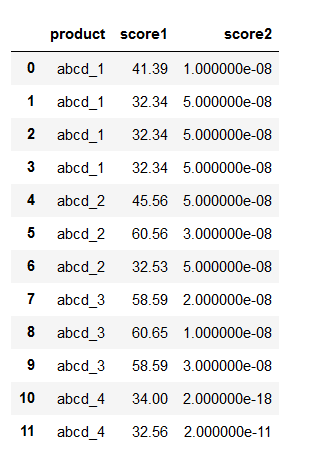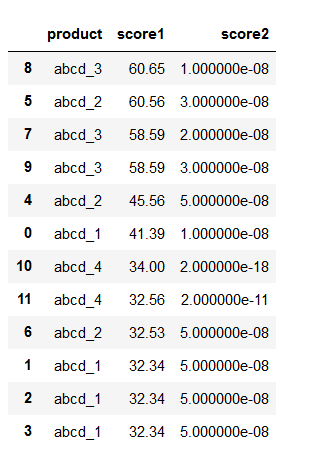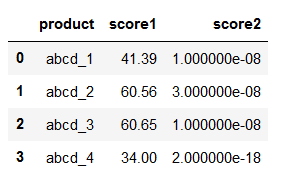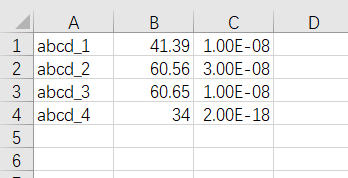2020-04-16 02:26

# 求问大神python排序问题

``````abcd_1   41.39    1.00E-08
abcd_1   32.34    5.00E-08
abcd_1   32.34    5.00E-08
abcd_1   32.34    5.00E-08
abcd_2   45.56    5.00E-08
abcd_2   60.56    3.00E-08
abcd_2   32.53    5.00E-08
abcd_3   58.59    2.00E-08
abcd_3   60.65    1.00E-08
abcd_3   58.59    3.00E-08
abcd_4   34.00    2.00E-18
abcd_4   32.56    2.00E-11
``````

• 写回答
• 好问题 提建议
• 追加酬金
• 关注问题
• 邀请回答

#### 2条回答默认 最新

•正在学C++ 2020-04-16 11:21
最佳回答
``````import pandas as pd
df
````````````df = df.sort_values(by=['score1','score2'],ascending=[False,True])   #False表示降序排序
df
````````````df = df.groupby('product',as_index=False).first()
# as_index=False表示使用原来的索引而不是以分组依据作为索引；first()表示保留第一个数据
df
````````````df.to_csv(path_or_buf='result.csv',header=None,index=None)